Anzeige

# CS369-FDM-FEM.pptx

courtoi
26. Mar 2023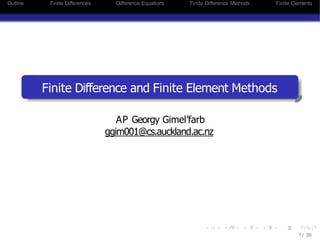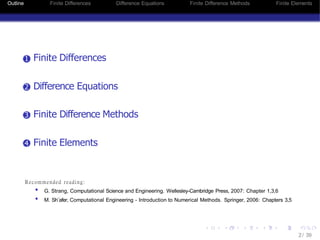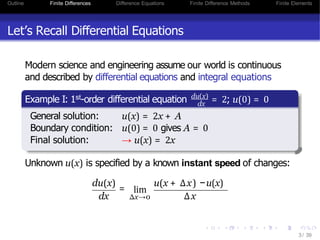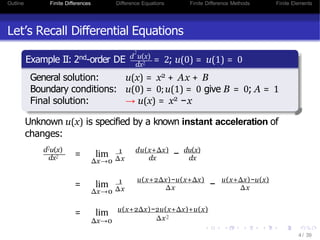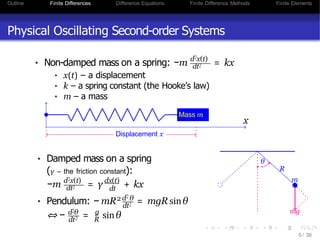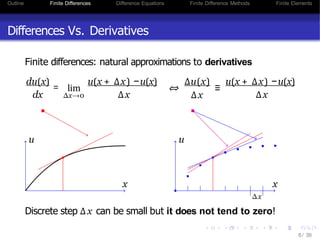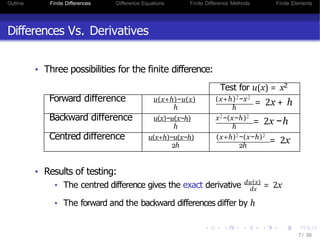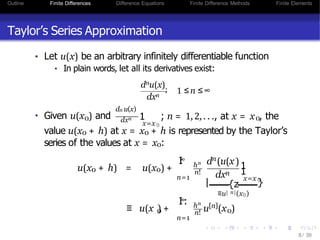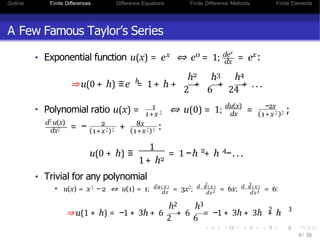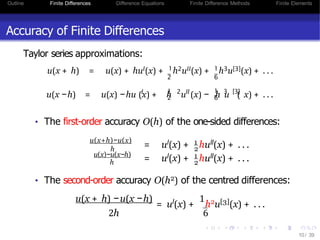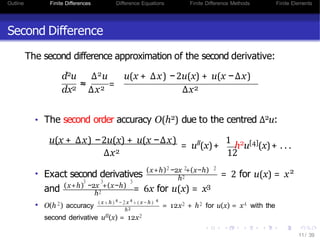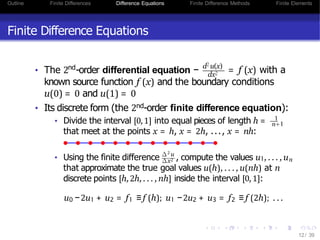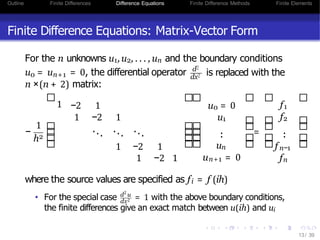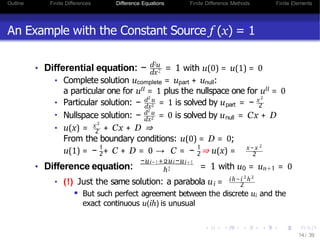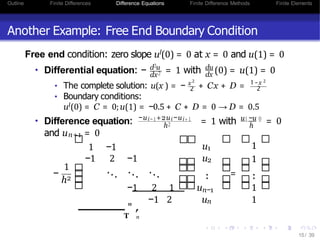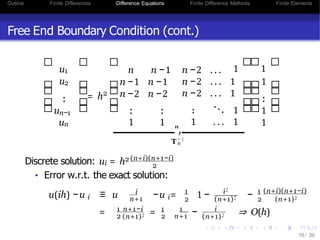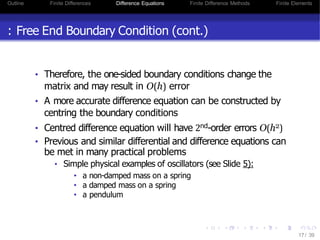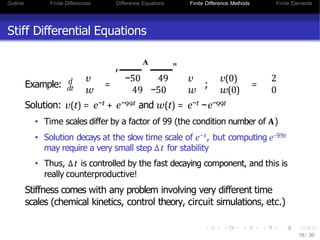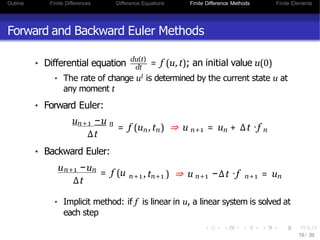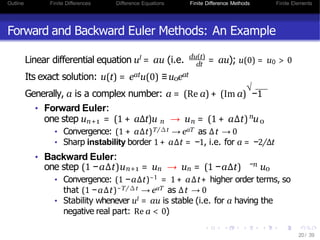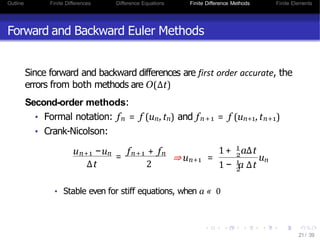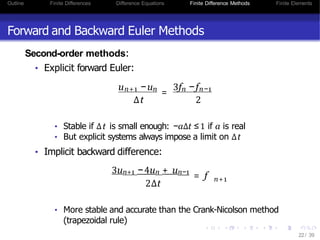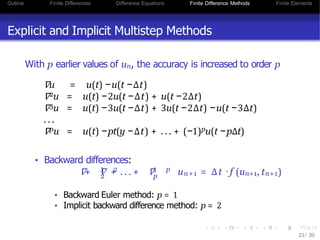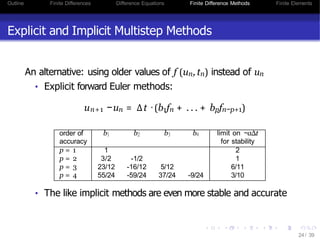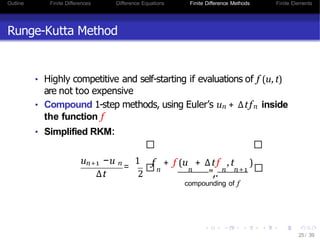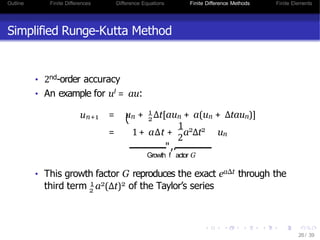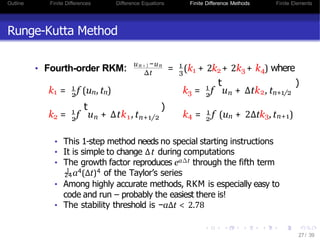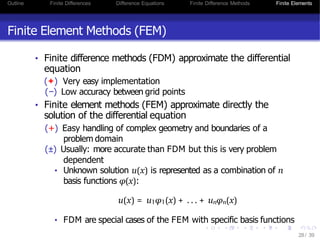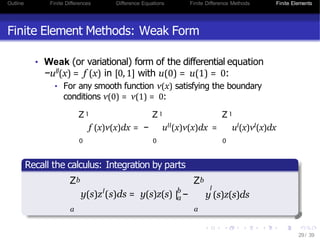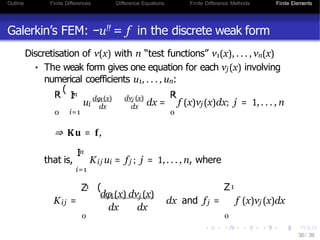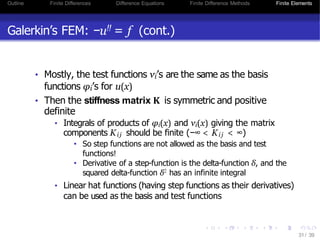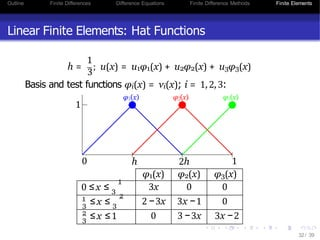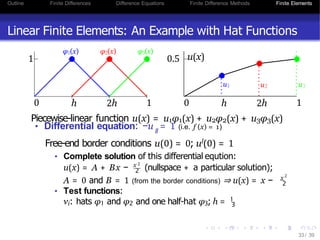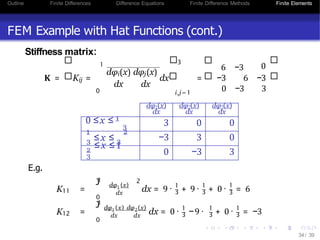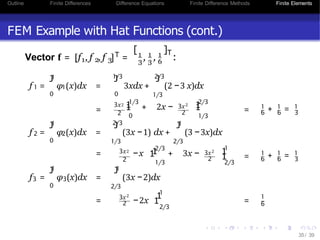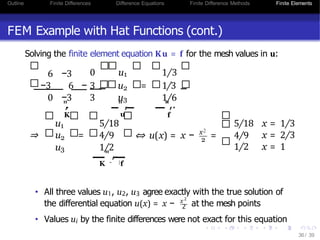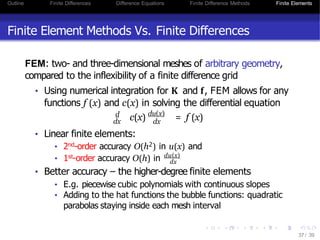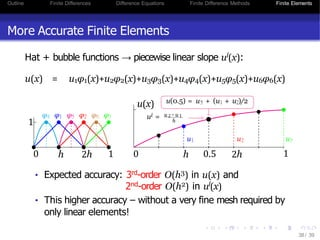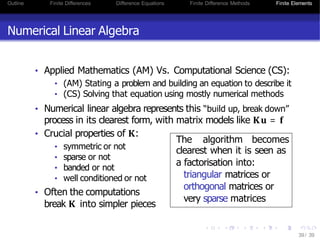1 von 39
Anzeige

### CS369-FDM-FEM.pptx

1. Outline Finite Differences Difference Equations Finite Difference Methods Finite Elements Finite Difference and Finite Element Methods 1/ 39 AP Georgy Gimel’farb ggim001@cs.auckland.ac.nz
2. Outline Finite Differences Difference Equations Finite Difference Methods Finite Elements 1 Finite Differences 2 Difference Equations 3 Finite Difference Methods 4 Finite Elements 2/ 39 Recommended reading: • G. Strang, Computational Science and Engineering. Wellesley-Cambridge Press, 2007: Chapter 1,3,6 • M. Sh¨afer, Computational Engineering - Introduction to Numerical Methods. Springer, 2006: Chapters 3,5
3. Outline Finite Differences Difference Equations Finite Difference Methods Finite Elements Let’s Recall Differential Equations Modern science and engineering assume our world is continuous and described by differential equations and integral equations dx Example I: 1st-order differential equation du(x) = 2; u(0) = 0 General solution: u(x) = 2x + A Boundary condition: u(0) = 0 gives A = 0 Final solution: → u(x) = 2x Unknown u(x) is specified by a known instant speed of changes: lim du(x) = u(x + ∆x) −u(x) dx ∆x→0 ∆x 3/ 39
4. Outline Finite Differences Difference Equations Finite Difference Methods Finite Elements Let’s Recall Differential Equations dx2 2 Example II: 2nd-order DE d u(x) = 2; u(0) = u(1) = 0 General solution: u(x) = x2 + Ax + B Boundary conditions: u(0) = 0;u(1) = 0 give B = 0; A = 1 Final solution: → u(x) = x2 −x Unknown u(x) is specified by a known instant acceleration of changes: d2u(x) dx2 4/ 39 = lim 1 ∆x→0 ∆x du(x+∆x) dx − du(x) dx = lim 1 ∆x→0 ∆x u(x+2∆x)−u(x+∆x) ∆x − u(x+∆x)−u(x) ∆x = lim ∆x→0 u(x+2∆x)−2u(x+∆x)+u(x) ∆x2
5. Outline Finite Differences Difference Equations Finite Difference Methods Finite Elements Physical Oscillating Second-order Systems d2x(t) • Non-damped mass on a spring: −m dt2 = kx • x(t) – a displacement • k – a spring constant (the Hooke’s law) • m – a mass x Mass m Displacement x • Damped mass on a spring (γ – the friction constant): d2x(t) dx(t) dt −m dt2 = γ + kx • Pendulum: − 2 d2 θ dt2 mR = mgRsinθ 2 d2θ g dt R ⇔ − = sinθ θ R m mg 5/ 39
6. Outline Finite Differences Difference Equations Finite Difference Methods Finite Elements Differences Vs. Derivatives Finite differences: natural approximations to derivatives dx ∆x→0 du(x) = u(x + ∆x) −u(x) ∆x lim ⇔ ∆u(x) ∆x ≡ u(x + ∆x) −u(x) ∆x u u x x ∆x Discrete step ∆x can be small but it does not tend to zero! 6/ 39
7. Outline Finite Differences Difference Equations Finite Difference Methods Finite Elements Differences Vs. Derivatives • Three possibilities for the finite difference: Test for u(x) = x2 Forward difference u(x+h)−u(x) h (x+h)2−x2 = 2x + h h Backward difference u(x)−u(x−h) h x2−(x−h)2 = 2x −h h Centred difference u(x+h)−u(x−h) 2h (x+h)2−(x−h)2 = 2x 2h 7/ 39 • Results of testing: dx • The centred difference gives the exact derivative du(x) = 2x • The forward and the backward differences differ by h
8. Outline Finite Differences Difference Equations Finite Difference Methods Finite Elements Taylor’s Series Approximation • Let u(x) be an arbitrary infinitely differentiable function • In plain words, let all its derivatives exist: dxn dnu(x) ; 1 ≤ n ≤ ∞ • 0 Given u(x ) and n x=x 0 0 d u(x) dxn 1 ; n = 1,2,. . ., at x = x , the value u(x0 + h) at x = x0 + h is represented by the Taylor’s series of the values at x = x0: u(x0 + h) = u(x0) + ∞ I : n=1 h n! n n d (u(x) dxn 1 1 x=x 0 | } ≡u[ { n z ](x0) 0 ≡ u(x ) + ∞ I: n=1 n n! 8/ 39 h [n] 0 u (x )
9. Outline Finite Differences Difference Equations Finite Difference Methods Finite Elements A Few Famous Taylor’s Series x 0 dex dx x • Exponential function u(x) = e ⇔ e = 1; = e : h h2 h3 h4 ⇒ u(0 + h) ≡ e = 1 + h + 2 + 6 + 24 + . . . • Polynomial ratio u(x) = 1 1+x 2 ⇔ u(0) = 1; du(x) dx = −2x (1+x ) 2 2 ; 2 d u(x) dx2 = − + 2 8x (1+x2)2 (1+x2)3 : u(0 + h) ≡ 1 1 + h2 2 4 = 1−h + h −. . . • Trivial for any polynomial 2 dx dx2 3 dx3 • u(x) = x3 − 2 ⇔ u(1) = 1; du(x) = 3x2; d u ( x ) = 6x; d u ( x ) = 6: h2 h3 ⇒ u(1 + h) = −1 + 3h + 6 2 + 6 6 = −1 + 3h + 3h + h 9/ 39 2 3
10. Outline Finite Differences Difference Equations Finite Difference Methods Finite Elements Accuracy of Finite Differences Taylor series approximations: u(x + h) = u(x) + hul(x) + 1h2ull(x) + 1h3u(x) + . . . 2 6 2 u(x −h) = u(x) −hu (x) + h l 1 2 ll 6 1 3  u (x) − h u ( x) + . . . • The first-order accuracy O(h) of the one-sided differences: u(x+h)−u(x) 2 = ul(x) + 1 hull(x) + . . . h u(x)−u(x−h) h 2 = ul(x) + 1 hull(x) + . . . • The second-order accuracy O(h2) of the centred differences: u(x + h) −u(x −h) = ul(x) + 1 h2u(x) + . . . 2h 6 10/ 39
11. Outline Finite Differences Difference Equations Finite Difference Methods Finite Elements ∆x2 Second Difference The second difference approximation of the second derivative: d2u ∆2u u(x + ∆x) −2u(x) + u(x −∆x) dx2 ≈ ∆x2 = ∆x2 • The second order accuracy O(h2) due to the centred ∆2u: u(x + ∆x) −2u(x) + u(x −∆x) = ull(x)+ 1 h2u(x)+ . . . 12 = 2 for u(x) = x • Exact second derivatives (x+h) −2x +(x−h) 2 2 2 h2 2 h2 3 3 3 and (x+h) −2x +(x−h) = 6x for u(x) = x3 2 • O(h ) accuracy 4 ( x + h ) 4 − 2 x 4 + ( x − h ) h2 11/ 39 = 12x2 + h2 for u(x) = x4 with the second derivative ull(x) = 12x2
12. Outline Finite Differences Difference Equations Finite Difference Methods Finite Elements Finite Difference Equations nd • The 2 -order differential equation − 2 d u(x) dx2 = f (x) with a known source function f (x) and the boundary conditions u(0) = 0 and u(1) = 0 • Its discrete form (the 2nd-order finite difference equation): • Divide the interval [0,1] into equal pieces of length h = 1 n+1 that meet at the points x = h, x = 2h, ..., x = nh: 2 ∆ u ∆x2 12/ 39 1 • Using the finite difference , compute the values u , . . . , un that approximate the true goal values u(h), . . . , u(nh) at n discrete points [h,2h, . . . , nh] inside the interval [0,1]: u0 −2u1 + u2 = f1 ≡ f (h); u1 −2u2 + u3 = f2 ≡ f (2h); . . .
13. Outline Finite Differences Difference Equations Finite Difference Methods Finite Elements Finite Difference Equations: Matrix-Vector Form 0 n+1 u = u = 0, the differential operator For the n unknowns u1,u2, . . . , un and the boundary conditions d2 dx2 is replaced with the n ×(n + 2) matrix: − 1 2 1 h −2 1 1 −2 1 ... ... ... 1 −2 1 1 −2 1 u0 = 0 u1 . . un un+1 = 0 = f1 f2 . . f n−1 fn where the source values are specified as fi = f (ih) 2 d u dx2 13/ 39 • For the special case = 1 with the above boundary conditions, the finite differences give an exact match between u(ih) and ui
14. Outline Finite Differences Difference Equations Finite Difference Methods Finite Elements An Example with the Constant Source f (x) = 1 d2u dx2 • Differential equation: − = 1 with u(0) = u(1) = 0 • Complete solution ucomplete = upart + unull: a particular one for ull = 1 plus the nullspace one for ull = 0 d u dx2 part x 2 2 2 2 d u dx2 null Nullspace solution: − = 0 is solved by u = Cx + D x2 2 • Particular solution: − = 1 is solved by u = − • • u(x) = + Cx + D ⇒ From the boundary conditions: u(0) = D = 0; 2 u(1) = − + C + D = 0 → 1 1 2 C = − ⇒ u(x) = 2 x − x 2 • Difference equation: −ui−1+2ui−ui+1 h2 = 1 with u0 = un+1 = 0 i • (!) Just the same solution: a parabola u = i h −i h 2 2 2 14/ 39 • But such perfect agreement between the discrete ui and the exact continuous u(ih) is unusual
15. Outline Finite Differences Difference Equations Finite Difference Methods Finite Elements Another Example: Free End Boundary Condition Free end condition: zero slope ul(0) = 0 at x = 0 and u(1) = 0 • dx2 d2u du dx Differential equation: − = 1 with (0) = u(1) = 0 • The complete solution: u(x x2 2 ) = − + Cx + D = 2 1−x 2 • Boundary conditions: ul(0) = C = 0;u(1) = −0.5 + C + D = 0 → D = 0.5 • Difference equation: = 1 with 1 0 h = 0 and un+1 = 0 − 1 h2 1 −1 −1 2 −1 ... ... ... −1 2 −ui−1+2ui−ui+1 u −u h2 15/ 39 u1 u2 −1 2 1 u T " , . n n−1 un = 1 . . . . 1 1 1
16. Outline Finite Differences Difference Equations Finite Difference Methods Finite Elements Free End Boundary Condition (cont.) u1 u2 . u . n−1 un = h2 n n −1 n −1 n −1 n −2 n −2 . . . 1 . 1 . ... ",. T − 1 n n −2 . . . n −2 . . . 1 1 n −2 . . . 1 . 1 1 . . . 1 . . 1 1 1 1 Discrete solution: ui = h2 (n+i)(n+1−i) 2 • Error w.r.t. the exact solution: i u(ih) −u ≡ u i n+1 i −u = 1 2 1 − i2 (n+1)2 16/ 39 − 1 2 (n+i)(n+1−i) (n+1)2 = 1 n+1−i 1 = − 1 i 2 (n+1)2 2 n+1 (n+1)2 ⇒ O(h)
17. Outline Finite Differences Difference Equations Finite Difference Methods Finite Elements : Free End Boundary Condition (cont.) • Therefore, the one-sided boundary conditions change the matrix and may result in O(h) error • A more accurate difference equation can be constructed by centring the boundary conditions • Centred difference equation will have 2nd-order errors O(h2) • Previous and similar differential and difference equations can be met in many practical problems • Simple physical examples of oscillators (see Slide 5): • a non-damped mass on a spring • a damped mass on a spring • a pendulum 17/ 39
18. Outline Finite Differences Difference Equations Finite Difference Methods Finite Elements Stiff Differential Equations Example: d dt = A , . " 18/ 39 v −50 49 v w 49 −50 w ; v(0) w(0) = 2 0 Solution: v(t) = e−t + e−99t and w(t) = e−t −e−99t • Time scales differ by a factor of 99 (the condition number of A) • Solution decays at the slow time scale of e−t, but computing e−99t may require a very small step ∆t for stability • Thus, ∆t is controlled by the fast decaying component, and this is really counterproductive! Stiffness comes with any problem involving very different time scales (chemical kinetics, control theory, circuit simulations, etc.)
19. Outline Finite Differences Difference Equations Finite Difference Methods Finite Elements Forward and Backward Euler Methods • Differential equation du(t) dt 19/ 39 = f (u, t); an initial value u(0) • The rate of change ul is determined by the current state u at any moment t • Forward Euler: u −u n+1 n ∆t = f (u , t ) ⇒ u = u + ∆t ·f n n n+1 n n • Backward Euler: ∆t un+1 −un = f (u n+1 n+1 , t ) ⇒ u n+1 −∆t ·f = u n+1 n • Implicit method: if f is linear in u, a linear system is solved at each step
20. Outline Finite Differences Difference Equations Finite Difference Methods Finite Elements Forward and Backward Euler Methods: An Example dt Linear differential equation ul = au (i.e. du(t) = au); u(0) = u0 > 0 Its exact solution: u(t) = eatu(0) ≡ u0eat Generally, a is a complex number: a = (Re a) + (Im a) √ −1 • Forward Euler: 20/ 39 n one step u = (1 + a∆t)u → u = (1 + a∆t) u n+1 n n 0 • Convergence: (1 + a∆t)T/∆t → eaT as ∆t → 0 • Sharp instability border 1 + a∆t = −1, i.e. for a = −2/∆t • Backward Euler: −n one step (1 −a∆t)un+1 = un → un = (1 −a∆t) u0 • Convergence: (1 −a∆t)−1 = 1 + a∆t+ higher order terms, so that (1 −a∆t)−T/∆t → eaT as ∆t → 0 • Stability whenever ul = au is stable (i.e. for a having the negative real part: Re a < 0)
21. Outline Finite Differences Difference Equations Finite Difference Methods Finite Elements Forward and Backward Euler Methods Since forward and backward differences are first order accurate, the errors from both methods are O(∆t) Second-order methods: • Formal notation: fn = f (un, tn) and fn+1 = f (un+1, tn+1) • Crank-Nicolson: 21/ 39 un+1 −un = fn+1 + fn ∆t 2 ⇒ un+1 = 1 2 1 + a∆t 1 2 1 − a ∆t un • Stable even for stiff equations, when a « 0
22. Outline Finite Differences Difference Equations Finite Difference Methods Finite Elements 2∆t 22/ 39 Forward and Backward Euler Methods Second-order methods: • Explicit forward Euler: un+1 −un = 3fn −fn−1 ∆t 2 • Stable if ∆t is small enough: −a∆t ≤ 1 if a is real • But explicit systems always impose a limit on ∆t • Implicit backward difference: 3un+1 −4un + un−1 = f n+1 • More stable and accurate than the Crank-Nicolson method (trapezoidal rule)
23. Outline Finite Differences Difference Equations Finite Difference Methods Finite Elements Explicit and Implicit Multistep Methods With p earlier values of un, the accuracy is increased to order p ∇u = u(t) −u(t −∆t) ∇2u = u(t) −2u(t −∆t) + u(t −2∆t) ∇3u = u(t) −3u(t −∆t) + 3u(t −2∆t) −u(t −3∆t) . . . ∇pu = u(t) −pt(y −∆t) + . . . + (−1)pu(t −p∆t) 23/ 39 • Backward differences: 2 p 1 2 1 p ∇+ ∇ + . . . + ∇ un+1 = ∆t ·f (un+1, tn+1) • Backward Euler method: p = 1 • Implicit backward difference method: p = 2
24. Outline Finite Differences Difference Equations Finite Difference Methods Finite Elements Explicit and Implicit Multistep Methods An alternative: using older values of f (un, tn) instead of un • Explicit forward Euler methods: un+1 −un = ∆t ·(b1fn + . . . + bpfn−p+1) 24/ 39 order of accuracy b1 b2 b3 b4 limit on −a∆t for stability p = 1 1 2 p = 2 3/2 -1/2 1 p = 3 23/12 -16/12 5/12 6/11 p = 4 55/24 -59/24 37/24 -9/24 3/10 • The like implicit methods are even more stable and accurate
25. Outline Finite Differences Difference Equations Finite Difference Methods Finite Elements Runge-Kutta Method • Highly competitive and self-starting if evaluations of f (u, t) are not too expensive • Compound 1-step methods, using Euler’s un + ∆tfn inside the function f • Simplified RKM: n+1 n u −u = 1 ∆t 2 25/ 39 n n n n+1 ",. compounding of f f + f (u + ∆tf , t )
26. Outline Finite Differences Difference Equations Finite Difference Methods Finite Elements Simplified Runge-Kutta Method • 2nd-order accuracy • An example for ul = au: un+1 2 = un + 1 ∆t[aun + a(un + ∆taun)] 1 ( = 1 + a∆t + 2 a2∆t2 un Growth " f ,. actor G • This growth factor G reproduces the exact ea∆t through the 26/ 39 2 third term 1 a2(∆t)2 of the Taylor’s series
27. Outline Finite Differences Difference Equations Finite Difference Methods Finite Elements Runge-Kutta Method • Fourth-order RKM: 27/ 39 un+1−un ∆t 1 3 = (k + 2 1 2 3 4 k + 2k + k ) where 2 k1 = 1 f (un, tn) 3 1 2 t k = f un + ∆tk2, tn+1/2 ) 2 1 t k = f un 1 n+1/2 ) 2 2 + ∆tk , t k4 = 1 f (un + 2∆tk3, tn+1) • This 1-step method needs no special starting instructions • It is simple to change ∆t during computations • The growth factor reproduces ea∆t through the fifth term 1 a4(∆t)4 of the Taylor’s series 24 • Among highly accurate methods, RKM is especially easy to code and run – probably the easiest there is! • The stability threshold is −a∆t < 2.78
28. Outline Finite Differences Difference Equations Finite Difference Methods Finite Elements Finite Element Methods (FEM) • Finite difference methods (FDM) approximate the differential equation (+) Very easy implementation (−) Low accuracy between grid points • Finite element methods (FEM) approximate directly the solution of the differential equation (+) Easy handling of complex geometry and boundaries of a problem domain (±) Usually: more accurate than FDM but this is very problem dependent • Unknown solution u(x) is represented as a combination of n basis functions φ(x): u(x) = u1φ1(x) + . . . + unφn(x) • FDM are special cases of the FEM with specific basis functions 28/ 39
29. Outline Finite Differences Difference Equations Finite Difference Methods Finite Elements Finite Element Methods: Weak Form • Weak (or variational) form of the differential equation −ull(x) = f (x) in [0,1] with u(0) = u(1) = 0: • For any smooth function ν(x) satisfying the boundary conditions ν(0) = ν(1) = 0: Z1 Z1 Z1 f (x)ν(x)dx = − ull(x)ν(x)dx = ul(x)νl(x)dx 0 0 0 Recall the calculus: Integration by parts Zb a 29/ 39 l y(s)z (s)ds = y(s)z(s) b a Zb a l | − y (s)z(s)ds
30. Outline Finite Differences Difference Equations Finite Difference Methods Finite Elements Galerkin’s FEM: −u!! = f in the discrete weak form Discretisation of ν(x) with n “test functions” ν1(x), . . . , νn(x) • The weak form gives one equation for each νj(x) involving numerical coefficients u1, . . . , un: 30/ 39 0 i=1 ui i dφ (x) dx ( j dν (x) dx 1 n 1 R I : R 0 dx = f (x)νj(x)dx; j = 1,. . . , n ⇒ Ku = f, n I : i=1 that is, Kij ui = fj ; j = 1,. . . , n, where Kij = 1 i j dφ (x) dν (x) dx dx Z ( 0 dx and fj = f (x)νj (x)dx 0 Z1
31. Outline Finite Differences Difference Equations Finite Difference Methods Finite Elements Galerkin’s FEM: −u!! = f (cont.) • Mostly, the test functions νi’s are the same as the basis functions φi’s for u(x) • Then the stiffness matrix K is symmetric and positive definite • Integrals of products of φi(x) and νi(x) giving the matrix components Ki j should be finite (−∞ < Ki j < ∞) • So step functions are not allowed as the basis and test functions! • Derivative of a step-function is the delta-function δ, and the squared delta-function δ2 has an infinite integral • Linear hat functions (having step functions as their derivatives) can be used as the basis and test functions 31/ 39
32. Outline Finite Differences Difference Equations Finite Difference Methods Finite Elements Linear Finite Elements: Hat Functions 1 h = 3 ; u(x) = u1φ1(x) + u2φ2(x) + u3φ3(x) Basis and test functions φi(x) = νi(x); i = 1,2,3: 0 h 2h 1 1 φ1(x) φ2(x) φ3(x) 32/ 39 φ1(x) φ2(x) φ3(x) 0 1 ≤ x ≤ 3 3x 0 0 1 3 2 ≤ x ≤ 3 2 −3x 3x −1 0 2 3 ≤ x ≤ 1 0 3 −3x 3x −2
33. Outline Finite Differences Difference Equations Finite Difference Methods Finite Elements Linear Finite Elements: An Example with Hat Functions 1 1 0.5 φ1(x) φ2(x) φ3(x) u(x) u2 u1 u3 0 h 2h 1 0 h 2h Piecewise-linear function u(x) = u1φ1(x) + u2φ2(x) + u3φ3(x) • Differential equation: −u = 1 (i.e. f (x) = 1) ll u(x) = A + Bx − x Free-end border conditions u(0) = 0; ul(0) = 1 • Complete solution of this differential eqution: 2 2 (nullspace + a particular solution); A = 0 and B = 1 (from the border conditions) ⇒ u(x) = x − x 2 2 • Test functions: νi: hats φ1 and φ2 and one half-hat φ3; h = 1 3 33/ 39
34. Outline Finite Differences Difference Equations Finite Difference Methods Finite Elements FEM Example with Hat Functions (cont.) Stiffness matrix: K = Kij = 1 0 dx dφi(x) dφj(x) dx dx 3 i,j=1 6 −3 = −3 6 −3 0 −3 3 0 dφ1(x) dx dφ2(x) dx dφ3(x) dx 0 ≤x ≤ 1 3 3 0 0 1 2 3 ≤ x ≤ 3 −3 3 0 ≤ x ≤ 1 2 3 0 −3 3 E.g. K11 = 1 J 0 1 dφ (x) dx 2 1 1 3 3 dx = 9 · + 9 · + 0 · 1 3 = 6 K12 = 1 J 0 dx 1 2 dφ (x) dφ (x) dx 3 1 1 3 1 3 34/ 39 dx = 0 · −9 · + 0 · = −3
35. Outline Finite Differences Difference Equations Finite Difference Methods Finite Elements FEM Example with Hat Functions (cont.) 1 2 3 Vector f = [f , f , f ] = , T 1 1 1 3 3 6 [ ]T , : J 0 1 1 f = φ (x)dx = 1 1/3 J 0 2/3 J 1/3 3xdx + (2 −3 x)dx = 2 1 1/3 0 3x2 2 1 3x 1 + 2x − 1 2 2/3 1/3 = 1 + 1 = 1 6 6 3 1 J 0 2 2 f = φ (x)dx = 2/3 J 1/3 (3x −1) 1 J 2/3 dx + (3 −3x)dx 2 1 2/3 1/3 = 3x −x 1 + 3x − 2 2 3x 2 1 1 1 2/3 = 1 + 1 = 1 6 6 3 1 J 0 f3 = φ3(x)dx = 1 J 2/3 (3x −2)dx = 2 3x 2 1 −2x 1 1 2/3 = 1 6 35/ 39
36. Outline Finite Differences Difference Equations Finite Difference Methods Finite Elements FEM Example with Hat Functions (cont.) Solving the finite element equation Ku = f for the mesh values in u: 6 −3 −3 6 − 0 −3 3 " , . K 2 u 3 u = 1/3 u3 1/6 0 u1 1/3 " , . ",. f u1 2 u3 5/18 ⇒ u = 4/ 1/2 K " − ,. 1f 9 ⇔ u( x2 2 5/18 x) = x − = 4/9 x = 1/3 x = 2/3 1/2 x = 1 • All three values u1, u2, u3 agree exactly with the true solution of x 2 2 36/ 39 the differential equation u(x) = x − at the mesh points • Values ui by the finite differences were not exact for this equation
37. Outline Finite Differences Difference Equations Finite Difference Methods Finite Elements Finite Element Methods Vs. Finite Differences FEM: two- and three-dimensional meshes of arbitrary geometry, compared to the inflexibility of a finite difference grid • Using numerical integration for K and f, FEM allows for any 37/ 39 functions f (x) and c(x) in solving the differential equation d dx c(x) du(x) dx = f (x) • Linear finite elements: • 2nd-order accuracy O(h2) in u(x) and • 1st-order accuracy O(h) in du(x) dx • Better accuracy – the higher-degree finite elements • E.g. piecewise cubic polynomials with continuous slopes • Adding to the hat functions the bubble functions: quadratic parabolas staying inside each mesh interval
38. Outline Finite Differences Difference Equations Finite Difference Methods Finite Elements More Accurate Finite Elements Hat + bubble functions → piecewise linear slope ul(x): u(x) = u1φ1(x)+u2φ2(x)+u3φ3(x)+u4φ4(x)+u5φ5(x)+u6φ6(x) 1 φ4 φ1 φ5 φ2 φ6 φ3 1 u(x) u(0.5) = u5 + (u1 + u2)/2 ul = u 2 − u 1 h u3 38/ 39 u1 u2 0 h 2h 1 0 h 0.5 2h • Expected accuracy: 3rd-order O(h3) in u(x) and 2nd-order O(h2) in ul(x) • This higher accuracy – without a very fine mesh required by only linear elements!
39. Outline Finite Differences Difference Equations Finite Difference Methods Finite Elements Numerical Linear Algebra • Applied Mathematics (AM) Vs. Computational Science (CS): • (AM) Stating a problem and building an equation to describe it • (CS) Solving that equation using mostly numerical methods • Numerical linear algebra represents this “build up, break down” process in its clearest form, with matrix models like Ku = f 39/ 39 • Crucial properties of K: • symmetric or not • sparse or not • banded or not • well conditioned or not • Often the computations break K into simpler pieces The algorithm becomes clearest when it is seen as a factorisation into: triangular matrices or orthogonal matrices or very sparse matrices
Anzeige